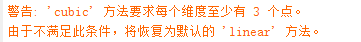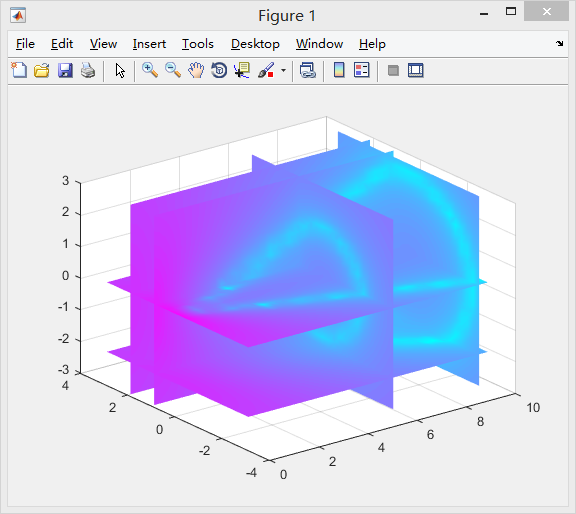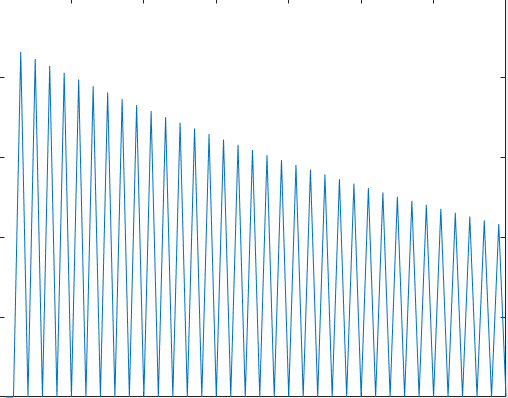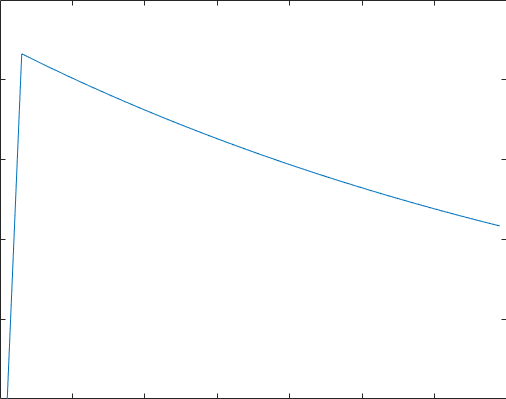• 新人请教大家一个关于matlab中函数interp3的问题，拜托指点一二。。 - 仿真模拟 - 小木虫 - 学术 科研 互动...
2021-04-22 14:50:10

困死了，先这样。

如果x，y的数据是二维的(楼主的数据)，只要用interp2就可以了。。

clc;clear;close all;

x=[10 20 30 40];%相当于以10为步进，取4个点

y=[5 15 25 35];

z=[10 23 33 41;07 17 26 37;10 20 30 40;08 18 31 43;]; %z必须为4x4矩阵

%v=[10 23 33 41;07 17 26 37;10 20 30 40;08 18 31 43;];

surf(x,y,z)

title('Original data Plot');

xlabel('X'), ylabel('Y'), zlabel('Z'),

colormap; colorbar;axis([0 50 0 40 0 50]);

xi = [1:1:40];%以1为步进，取点

yi = [5:1:35];

[x2,y2]=meshgrid(xi,yi);%参考help案例产生新的xyz

t11=interp2(x,y,z,x2,y2,'cubic');

figure(2)

surf(xi,yi,t11)

title('After Fit data Plot');

xlabel('X'), ylabel('Y'), zlabel('Z'),

colormap, colorbar;axis([0 50 0 40 0 50]);，

更多相关内容
• ba_interp3 是 3D 体积的快速插值例程。 它可以用作 matlabs interp3 函数的替代品。 它支持最近邻、三线性和三三次插值。 限制：唯一的限制是，数据必须位于规则间隔的网格上（即来自 meshgrid. 增强功能： ba_...matlab
• splinterp 是一个轻量级、多线程、支持 MEX 的 C++ 标头模板库，用于并行计算线性、双线性和三线性插值。matlab
• 如题，MATLAB里面有interp1，interp2和interp3等等插值方法 也有‘linear’：线性插值（缺省算法）；‘cubic’：三次插值；‘spline’：三次样条插值；‘ nearest’：最邻近插值。等插值选择 但如何用两张图像去插...

如题，MATLAB里面有interp1，interp2和interp3等等插值方法

也有‘linear’：线性插值（缺省算法）； ‘cubic’：三次插值； ‘spline’：三次样条插值；‘ nearest’：最邻近插值。等插值选择

但如何用两张图像去插中间一张呢，或者用四张去插中间的一张呢

我们选择用interp3

input_path = './cremi/';
out_path = './cremi/0005_interp.png';
temp = zeros(1761,1680,4, 'uint8');

temp(:,:,1) = imread([input_path, num2str(3, '%04d'), '.png']);
temp(:,:,2) = imread([input_path, num2str(4, '%04d'), '.png']);
temp(:,:,3) = imread([input_path, num2str(6, '%04d'), '.png']);
temp(:,:,4) = imread([input_path, num2str(7, '%04d'), '.png']);

temp = double(temp);
[x,y,z] = size(temp);
[hx,hy,hz] = meshgrid(1:y, 1:x, 2:1/2:3);
hr = interp3(temp, hx, hy, hz, 'cubic');
imwrite(uint8(hr(:,:,2)), out_path)

注意：

cubic插值至少需要三张图像展开全文matlab
• 三次样条插值常常需要多个输入参数，这里提供两种方式的代码实现该算法。算法可以直接在VS上运行，以方便读者能够快速学习和理解三次样条插值。
• CUDA NetCDF Interp 该工具的目的是使用NVidia GPU加速3D数据插值。 目前，内核只是一个简单的三线性插值，但可能还会更多。 安装 使用install.sh使用CMake构建和安装。 默认情况下，这会将项目安装到build / bin中...C++
•  1、VI = interp3(X,Y,Z,V,XI,YI,ZI) %找出由参量X,Y,Z决定的三元函数V=V(X,Y,Z)在点（XI,YI,ZI）的值。参量XI,YI,ZI是同型阵列或向量。若向量参量XI,YI,ZI是不同长度，不同方向（行或列）的向量，这时输出参量VI与...

【语法说明】

1、VI = interp3(X,Y,Z,V,XI,YI,ZI) %找出由参量X,Y,Z决定的三元函数V=V(X,Y,Z)在点（XI,YI,ZI）的值。参量XI,YI,ZI是同型阵列或向量。若向量参量XI,YI,ZI是不同长度，不同方向（行或列）的向量，这时输出参量VI与Y1,Y2,Y3为同型矩阵。其中Y1,Y2,Y3为用命令meshgrid(XI,YI,ZI)生成的同型阵列。若插值点(XI,YI,ZI)中有位于点(X,Y,Z)之外的点，则相应地返回特殊变量值NaN

2、VI = interp3(V,XI,YI,ZI) %缺省地，X=1:N，Y=1:M，Z=1:P，其中，[M,N,P]=size(V)，再按上面的情形计算。

3、VI = interp3(V,n) %作n次递归计算，在V的每两个元素之间插入它们的三维插值。这样，V的阶数将不断增加。interp3(V)等价于interp3(V,1)。

4、VI = interp3(…,method) %用指定的算法method作插值计算：

‘linear’：线性插值（缺省算法）；

‘cubic’：三次插值；

‘spline’：三次样条插值；

‘  nearest’：最邻近插值。

说明 在所有的算法中，都要求X,Y,Z是单调且有相同的格点形式。当X,Y,Z是等距且单调时，用算法’*linear’，’*cubic’，’*nearest’，可得到快速插值。

【实例介绍】

[x,y,z,v] = flow(20);
>>[xx,yy,zz] = meshgrid(.1:.25:10, -3:.25:3, -3:.25:3);
>>vv = interp3(x,y,z,v,xx,yy,zz);
>>slice(xx,yy,zz,vv,[6 9.5],[1 2],[-2 .2]); shading interp;colormap cool展开全文• interp1 - 一元函数插值spline - 样条插值polyfit - 多项式插值或拟合lsqnonlin - 最小二乘法lsqcurvefit - 最小二乘拟合csape - 各种边界条件的样条插值csaps - 样条拟合interp2 - 二元函数插值griddata - 不规则...
• 与matlab结果一致的以为插值函数，结果完全一致。
• interp函数的实现需要调用filter函数（MATLAB内置），为此，我特地实现了自己的filter函数，可以从我上传的资源里面找到
• ## matlab interp插值函数

千次阅读 2019-11-20 22:48:24
什么是插值函数咧，我所理解的插值函数就是，有一些点但是直接plot出来的图，不大好看，可能是锯齿的散点的，但是你想要一个平滑...MATLAB中的插值函数为interp1，其调用格式为： yi= interp1(x,y,xi,'method') 其...

什么是插值函数咧，我所理解的插值函数就是，有一些点但是直接plot出来的图，不大好看，可能是锯齿的散点的，但是你想要一个平滑的曲线，而且去掉那些不大好看的点，这就需要插值函数来解决了。

MATLAB中的插值函数为interp1，其调用格式为： yi= interp1(x,y,xi,'method')

其中x，y为插值点，yi为在被插值点xi处的插值结果；x,y为向量， 'method'表示采用的插值方法，MATLAB提供的插值方法有几种： 'method'是最邻近插值， 'linear'线性插值； 'spline'三次样条插值； 'cubic'立方插值．缺省时表示线性插值。

注意：所有的插值方法都要求x是单调的，并且xi不能够超过x的范围。

用指定的算法计算插值：
’nearest’：最近邻点插值，直接完成计算；
’linear’：线性插值（缺省方式），直接完成计算；
’spline’：三次样条函数插值。
’cubic’：该方法保留单调性与数据的外形；图一因为有0值使其像个锯齿波，巨丑，而且也不适用某些仿真，插值过后顺眼多了，然后就是二维三维插值函数。

### 命令2 interp2

功能 二维数据内插值
(1)ZI = interp2(X,Y,Z,XI,YI,method)
返回矩阵ZI，其元素包含对应于参量XI 与YI（可以是向量、或同型矩阵） 的元素， 即Zi(i,j) ←[Xi(i,j),yi(i,j)]。用户可以输入行向量和列向量Xi 与Yi，此时，输出向量Zi 与矩阵meshgrid(xi,yi)是同型的。
(2)ZI = interp2(Z,XI,YI)
缺省地，X=1:n、Y=1:m，其中[m,n]=size(Z)。再按第一种情形进行计算。

用指定的算法method 计算二维插值：
’linear’：双线性插值算法（缺省算法）；
’nearest’：最临近插值；
’spline’：三次样条插值；
’cubic’：双三次插值。

[X,Y] = meshgrid(-3:.25:3);
Z = peaks(X,Y);
[XI,YI] = meshgrid(-3:.125:3);
ZZ = interp2(X,Y,Z,XI,YI);
surfl(X,Y,Z);hold on;
surfl(XI,YI,ZZ+15)
axis([-3 3 -3 3 -5 20]);shading flat
hold off

### 命令3 interp3

(4)VI = interp3(X,Y,Z,V,XI,YI,ZI,method)
找出由参量X,Y,Z决定的三元函数V=V(X,Y,Z)在点（XI,YI,ZI）的值。
%用指定的算法method 作插值计算：
‘linear’：线性插值（缺省算法）；
‘cubic’：三次插值；
‘spline’：三次样条插值；
‘nearest’：最邻近插值。

[x,y,z,v] = flow(20);

[xx,yy,zz] = meshgrid(.1:.25:10, -3:.25:3,
-3:.25:3);

vv = interp3(x,y,z,v,xx,yy,zz);

slice(xx,yy,zz,vv,[6 9.5],[1 2],[-2 .2]); shading interp;colormap cool

### 命令4 griddata

功能 数据格点
(1)ZI = griddata(x,y,z,XI,YI,method)
用二元函数z=f(x,y)的曲面拟合有不规则的数据向量x,y,z。griddata 将返回曲面z 在点（XI,YI）处的插值。曲面总是经过这些数据点（x,y,z）的输入参量（XI,YI）通常是规则的格点（像用命令meshgrid 生成的一样）。

用指定的算法method 计算：
‘linear’：基于三角形的线性插值（缺省算法）；
‘cubic’： 基于三角形的三次插值；
‘nearest’：最邻近插值法；
‘v4’：MATLAB 4 中的griddata 算法。

### 命令5 spline

功能 三次样条数据插值
格式
(1)yy = spline(x,y,xx)

x = [0 2 4 5 8 12 12.8 17.2 19.9 20]; y = exp(x).*sin(x);

xx = 0:.25:20;

yy = spline(x,y,xx);

plot(x,y,'o',xx,yy)

### 命令6 meshgrid

功能 生成用于画三维图形的矩阵数据
格式 [X,Y] = meshgrid(x,y) 将由向量x，y（可以是不同方向的）指定的区域[min(x)，max(x) ， min(y) ， max(y)] 用直线x=x(i),y=y(j) （ i=1,2,…,length(x) ，j=1,2,…,length(y)）进行划分。这样，得到了length(x)*length(y)个点，
这些点的横坐标用矩阵X 表示，X 的每个行向量与向量x 相同；这些点的纵坐标用矩阵Y 表示，Y 的每个列向量与向量y 相同。其中X,Y可用于计算二元函数z=f(x,y)与三维图形中xy 平面矩形定义域的划分或曲面作图。

[X,Y] = meshgrid(x) %等价于[X,Y]=meshgrid(x,x)。
[X,Y,Z] = meshgrid(x,y,z) %生成三维阵列X,Y,Z，用于计算三元函数v=f(x,y,z)或三维容积图。

[X,Y] = meshgrid(1:3,10:14)

展开全文• 句法vq = interp1gap(v) vq = interp1gap(x,v,xq) vq = interp1gap(...,maxgapval) vq = interp1gap(...,'方法') vq = interp1gap(...,'interpval',vval) vq = interp1gap(...,'extrap',extrapval) 描述vq = ...matlab
• python中实现了matlab中二维数据内插值interp2的方法。大家可以看一下。
• 主要介绍了matlab中二维插值函数interp2的使用详解，具有很好的参考价值，希望对大家有所帮助。一起跟随小编过来看看吧
• 主要介绍了python中的插值 scipy-interp的实现代码,需要的朋友可以参考下
• interp3(V)等价于interp3(V,1)。 VI=interp3(...,method)：用指定的算法method做插值计算。linear为线性插值（默认算法），cubic为三次插值，spline为三次样条插值，nearest为最邻近插值。 interpn函数——n维...
• 它的运行速度至少比“interp1q”快 3 倍，比“interp1”快 8 倍，并且随着 m=length(x) 的增加，速度提高了 10 倍以上（参见附加的性能图）。 与“ interp1q”一样，此功能不进行输入检查。 要正常工作，用户必须...matlab
• 我最近在matlab中用到插值法，看起来有interp1/2/3和interpn这两个函数可以用。然而，我惊讶地发现，这两类函数不是等价的： interp1和interpn是等价的，结果都是1584 interp1([1,2],[0,14317],1.1107)=1584 ...matlab
• 当我尝试查询新的[温度，压力]对时，例如[0.2,341]这样做 interp2(Temperature, Pressure, Cv, 0.2, 341) 我收到以下错误： Error using griddedInterpolant The grid vectors must be strictly monotonically ...
• matlab interp函数计算插值，不晓得对不对，看着玩玩吧
• scipy.interpolate.interp2d插值函数 ...Platform: Spyder 3 SciPy 1.5.0 在使用interp2d进行插值时，当输入的纵坐标顺序为正序时（递增序列，如[0, 1, 2, …10]），插值结果是正常的；而当输入逆序的纵坐标时（递减python scipy numpy
• 使用 Matlab 的 interp3 函数，我的计算机由于矢量化 interp3 的内存需求高而挂起。 所以我决定写一个 mex 文件来按顺序插入数据。 速度增益高达 5 倍。 当 interp3 设置为线性时，此函数充当 matlab 的 interp3 ...matlab
• interpinterpinterpinterpinterpinterpinterp 插值插值插值插值插值插值插值插值插值插值插值
• program main implicit none integer :: i real*8 :: x(9), y(9), t, z !// x与y的数据来源于matlab的interp1帮助... x = [0.d0,0.785398163397448d0,1.57079632679490d0,2.35619449019235d0,3.14159265358979d0.
• matlab中interp函数源码ALGLIB+ 这是 . ALGLIB+ 将提供静态库和一些类似于 MATLAB 函数的新函数，例如 interp1d、interp2d 等。 背景 当我用来开发热液系统模拟器时，必须使用插值函数来计算每个压力和温度点的流体...
• matlab开发-interp1nantablelookup。从表[x，y]中查找Xi，否则发现返回Y，No。
• Z3 Z3是Microsoft Research的一个定理证明者。它是根据获得。 如果您不熟悉Z3，则可以从开始。 Z3可以使用 ， 或构建。它提供。 有关Z3的各种稳定版本的，请参阅。 建置状态 Windows x64 Windows x86 ...请C++...